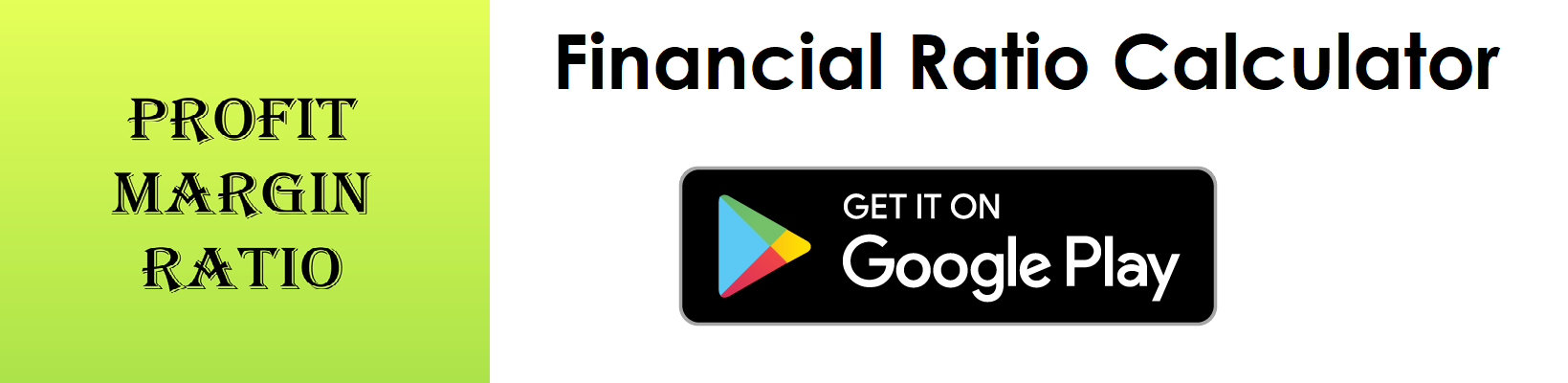# Debt Equity Ratio Calculator

Key in the Total Liabilities and Total Equity Values from your Balance Sheet to the respective fields given below and then click Calculate to get the desired result.

##### Debt to Equity Ratio Calculator - Glossary:
Using our debt to equity ratio calculator, helps to compare, measure, understand the overall health of the business.

##### Debt to Equity Ratio:
Shows the proportion of Total Liabilities to Total Equity. In simple words, it measures the riskiness of a company's financial structure.

##### How to calculate?
Formula: Debt to Equity Ratio = Total Liabilities/Total Equity
This is a balance sheet component; the values are commonly stated against Total Liabilities and Total Equity.

##### Total Liabilities:
Total Liabilities is the source of the funds such as short term and long term external borrowing that is mainly used to fund its asset purchase.

##### Total Equity:
Total equity is the amount invested by shareholders of the company.

##### Example:
Debt to equity (D/E) ratio for a company with a total liability of \$360,000 and total equity of \$720,000 is 0.5:1. It means that, the company has an exposure of 50% in the form of total liabilities to total equity. In other words, the assets of the company are funded 2-to-1 by investors to creditors.

# Download our Mobile Apps - today.